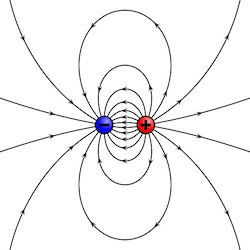## Estimating the Dipole Radiation Power using Dimensional AnalysisAn electric dipole is characterized by the dipole moment $d$ which depends on both the charge $e$ and a the separation $s$ (but not independently on each).
Estimating the power radiated by an oscillating electric dipole is an excellent example for the usage of dimensional analysis.

Let us assume we have a dipole oscillating with frequency $\omega$. The relevant equations to describe the problem are obviously Maxwell's equations. Thus, unlike the electrostatic description of the Hydrogen Atom, the speed of light $c$ will be relevant for the solution. Moreover, instead of an expression that depends on the electron charge $e$, we will seek one which depends on the dipole moment $d$, which is charge times displacement: $\left[d\right] = esu \cdot cm$. The expression will obviously depend on $\omega$ as well. It wont depend on $\hbar$ because the problem is not a quantum mechanical one. Likewise, the expression will also be independent of $G$ because the problems is unrelated to gravity.

Let us look at and the dimensionality of the relevant variables.

We seek an emitted power: $$\left[P\right] = {\mathrm{energy} \over \mathrm{unit time}} = {\mathrm{erg} \over \mathrm{s}} = {\mathrm{gr~cm^2} \over \mathrm{s^2}} {1 \over \mathrm{s}} = {\mathrm{gr~cm^2} \over \mathrm{s^3}}$$ The variables upon which it can depend are $$\left[c\right] = {\mathrm{cm} \over \mathrm{s}}, ~~~ \left[\omega\right]{ 1 \over \mathrm{s}}.$$ and the dipole moment: $$\left[d^2 \right] = \mathrm{esu}^2 \mathrm{cm}^2 = \mathrm{erg}\cdot \mathrm{cm^3} = {\mathrm{gr~cm^2} \over \mathrm{s^2}} \mathrm{cm}^3 = {\mathrm{gr~cm^5} \over \mathrm{s^2}}.$$ In order to have gr in the final result for $P$, we therefore need to take the first power of $d^2$. Thus $$\left[ {P \over d^2} \right] = {\mathrm{gr~cm^5} \over \mathrm{s^2}} { \mathrm{s^3} \over \mathrm{gr~cm^2}} = { 1\over \mathrm{s}~\mathrm{cm^3}}.$$ To get rid of the cm, we must multiply by $c^3$, and obtain: $$\left[{c^3 P \over d^2}\right] = { 1\over \mathrm{s}~\mathrm{cm^3}} {\mathrm{cm}^3 \over \mathrm{s}^3} = {1 \over \mathrm{s}^4}.$$ Last, by multiplying by $\omega^4$ we will get a dimensionless number: $$\left[{c^3 P \over d^2 \omega^4}\right] = 1.$$ Thus, to within a dimensionless constant, the power emitted is $$P \sim {d^2 \omega^4 \over c^3}.$$ To determine the actual prefactor it would be necessary to solve the full problem.

## Blogroll

Sensible Climate
Physics and more Other